Reach Us+44-7482-875032
A Mathematical Model in Reduction of Cost on Transportation of Sugarcane and the Loss Due To the Accident in Transportation | OMICS International
Journal of Applied & Computational Mathematics
All submissions of the EM system will be redirected to Online Manuscript Submission System. Authors are requested to submit articles directly to Online Manuscript Submission System of respective journal.

# A Mathematical Model in Reduction of Cost on Transportation of Sugarcane and the Loss Due To the Accident in Transportation

P. Saravanamoorthi1* and V. Navaneethakumar2

1Assistant Professor (Sr. Gr), Department of Mathematics, Bannari Amman Institute of Technology, Sathyamangalam, Tamilnadu, India

2Professor, Adhiyamaan College of Engineering, Hosur, Tamilnadu, India

*Corresponding Author:
P. Saravanamoorthi
Assistant Professor (Sr. Gr.), Department of Mathematics
Bannari Amman Institute of Technology
Sathyamangalam, Tamilnadu, India
E-mail: [email protected]

Received Date: January 26, 2012; Accepted Date: May 14, 2012; Published Date: May 18, 2012

Citation: Saravanamoorthi P, Navaneethakumar V (2012) A Mathematical Model in Reduction of Cost on Transportation of Sugarcane and the Loss Due To the Accident in Transportation. J Appl Computat Math 1:106. doi: 10.4172/2168-9679.1000106

Copyright: ©2012 Saravanamoorthi P, et al. This is an open-access article distributed under the terms of the Creative Commons Attribution License, which permits unrestricted use, distribution, and reproduction in any medium, provided the original author and source are credited.

Visit for more related articles at Journal of Applied & Computational Mathematics

#### Abstract

Sugarcane is a main economic crop to the farmers. The farmers, the people and the factories depend on this crop. Farmers who are cultivating the sugarcane have to send to their product to the nearest factories. Some vehicles are utilized for transporting the sugarcane to the factory. The vehicles are lorries, bullock carts or tractors. If the cost of transportation of sugarcane, sent to the factory is minimum, then the total processing cost will also be minimized. In this paper, the idea of minimum transportation cost is discussed using Linear Programming Problems and the mode of transportation between the field and the factory. The possibility of loss due to accident is also discussed in order to get ready for the sudden loss of the product.

#### Keywords

Sugarcane; Transportation of sugarcane; User optimization problem; Mode of transportation; Transportation cost; Road quality; Loss of the vehicles due to accident

90B06

#### Introduction

Transportation networks are complex, large scale systems and come in a variety of forms such as road, rail, air and water way networks. Transporattion networks provide the foundation for the functioning of our economics and socities through the movement of people, goods and services. From an economic perspective, the supply in such network systems is represented by the underlying network topology and the cost characteristics where the demand is represented by the users of the transportation systems. An equillibrium occurs when there is number of trips between the origin ( in this case, it is discussed as the transportation of sugarcane from various fields ) to the destination (here, it is defined as a factory where the sugarcane is processed for getting sugar). In the ancient period, the transportation and its control was studied. For example, Romans imposed controls over chariot traffic during different times of day in order to deal with the congestion . From an economic perspective, some of the earliest combinations to the subject data to Khol  and to Pigou , who considered a twonode two-line transportation network, identified congestion as a problem and recognised that distinct behavioural concepts regarding route selection might prevail .

The formal study of transportation networks has challenged transportation scientists, economists, operations researchers, engineers and physicists for reasons including the size and the scope of the systems involved; the behaviour of the users of the network which may vary according to the application setting, there by leading to different optimality concepts. According to estimates, Road congestion results in approximately 100 billion US dollars in lost productivity in the United States alone with the figure being about 150 billion US dollars in Europe with the number of cars expected to increase by 50 percent by 2010 and to double by 2030 . In particular, the growth in the usage of motorized vehicles especially in the developing world, is increasing in some countries such as China and India. Moreover, in many of todays’ transportation networks, the “non - cooperative” behaviour of users aggrevate the congestion problem.

Transportation concepts play an important role in Agricultural inTransportation concepts play an important role in Agricultural industry also. Transportation is used for the importing of raw materials from any source destinations and to sending of finished goods to the market etc. In recent trend, agricultural industry is also mechanised. Many of the works are done by machines but some works involve much costs. Sugarcane is the main economic crop to the farmers as well as the society. After harvesting the sugarcane, it should be transported to the factory nearer to the fields. But many farmers make an agreement with the factory to carry the products by their own risk. There are many routes identified and are known to the carrier. Here after, the product is called as sugarcane. Out of which, some routes may have certain damages and some of the routes are good in condition. If the vehicle uses the damaged route, the material will also get damaged because of overweight, the sugarcane will collide and as a result be crushed. Due to collision , the juice will be crushed out automatically. There will be a loss of weight of the sugarcane. Usually, the transporatation cost is calculated on the basis of the distance travelled between the source (Fields) and the destinations (Factory). But, if a route has some defects, there will be an additional loss for the goods (Sugarcane) and this loss should be incorporated with the transportation cost.

#### Fundamental Decision Making Models

Over a half century ago, Wardrop  explicitly considered alternative possible behaviours of the users of the transporation networks, notably, urban transportation networks and stated two principles, which are commonly named after him.

First principle : The jouney time of all routes actually used are equal and less than those which would be experienced by a single vehicle on any unused route.

Second Principle: The average time is minimal.

The principle corresponds to the behavioural principle in which travellers seek to (unilaterally) determine their minimal cost of travel whereas the second principle corresponds to the behavioural principle in which the total cost in the network is minimum. Beckmann et al.  were the first to rigorously formulate these conditions mathematically. Specifically, Beckmann et al.  established the equivallence between the transportation network equillibrium conditions, which state that all used paths connecting origin/destination pair will have equal and minimal travel times (corresponding to the Wardrop’s first principle), and the Khun and Tucker  conditions of an appropriately constructed optimization problem, under a symmetry assumption on the underlying functions . Hence, in this case, the equillibrium link and path flows could be obtained as the solution of a mathematical programming problem.

#### An Optimum Transportation Problem

This optimized network problem is also commonly referred to the transportation literature as the traffic assignment problem. Here, in transporting the sugarcane from the fields to the factory, many routes are known. This forms a transportation network. Consider a general network G = (N,L) where N denotes the set of nodes and L being the set of directed links. Links connect pairs of nodes in the network and are denoted by a,b,c... .Let p denotes a path from a field to the factory. This path should be acyclic in nature. So, there may be more than one path possible from the field to the factory concerned. Let Pω be the set of all paths related to the factory and the field.

ie., Pω = {p,q, r,.....}

Let Xp denotes the non negative flow on the path p and let fa denotes the flow on the link a. In sugarcane transportation, each field acting as origin and the path p is a path way between the field and the factory. There will be a non-negative flow of certain amount of sugarcane transported.This amount is specified as tons. If the prescribed tonnage is not met by the vehicle, it goes to the next origin (field) and collect the required tonnage of sugarcane and it will be sent to the factory.

Let fa may be defined as below

fa={ 1 if a ∈ p

0 if a ∉ p

Let the maximum tonnage of the vehicle be T . Different vehicles will have different tonnage capacities. For example, Lorries can carry a maximum amount of the sugarcane , Tractors can take the sugarcane lesser than the lorries and the Bullock carts can take only the least amount of sugarcane to the factory from the fields concerned. As far as the vehicles are concerned, their optimization network can be defined as user optimization problem. The user of the transportation facility is interested to reduce the transportation cost as well as the loss incurred to the sugarcane during the transportation.

Optimization procedure of the user-optimization problems

Always the transportation cost is determined by the distance between the factory and the field. So, the objective of the transportation problem is to reduce the transportation cost along the route specified or required for transportation.Let Cai fai be the cost of transportation along the route selected between the factory and the field where i denotes the ith field . Let n1 , n2 , n3 , ..... be the number of units of sugarcane harvested in the fields 1, 2 , ....... m respectively.

Let N be the maximum sugarcane manufactured in an area and then the Linear programming problem is given by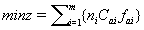subject to the constraint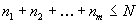The quality of the road may be considered for transportation. Some routes have less cost of transportation but they are very bad in condition for tranasportation. If the transportation is made through the route, quality of the sugarcane also gets damaged. If the quality is lost, the loss in the sugarcane will have to be calculated.The calculation of total ransportation cost is the inclusion of transportation cost of the vehicle and the amount lost due to loss in the sugarcane in selecting a tough route. To use a mathematical model, the quality of the road is considered by finding the probability or the possibility of the sugarcane that gets damaged. Let pi be the probability of loss through the route and the number of units of loss to the sugarcane be considered as p(xi). Let C be the cost of one unit of sugarcane and the total loss is given by C*p(xi) . Here, the cost of sugarcane is usually fixed per ton. The total loss through all the routes is equal to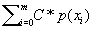where i =1,2,3,........ and they are the origins or the fields from where the transportation of sugarcane is made to the factory.

Then the objective function and their corresponding constraints are given below.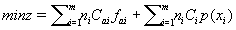subject to the constraints: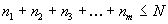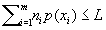(L- loss permitted at the maximum) ni ≥0 , Cai*fai≥ 0 , N ≥ 0 , p(xi) ≥0. Here, all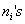are variables. This problem gives number of tons of sugarcane transportaed through the routes in such a way that the total transportation cost is minimum with a minimum loss in the sugarcane.

Numerical example

Consider the origins (where the fields are located) Numbiyur and Pulliampatti. The route from Numbiyur via i. Kurumandur, Sathy ii. Kadathur, Ariyappampalayam and Sathy. Let the route through Kurumandur be denoted as AR1 and via Kadathur be assumed as AR2 . And the second origin is assumed, here, is from Pulliampatti. The following are the notations assumed to the routes known. The route through Kadathur is BR1 and via Ariyappampalayam is taken as BR2 . Let there be 2000 tons of Sugarcane to be transported to the factory from the fields in a particular area (Table 1).

 Route Distance Cost of Transportation Probability of Loss Total Cost in kms in Rs per unit AR1 30 37 0.01 0.37 AR2 24 29 0.09 2.61 BR1 28 32 0.08 2.56 BR2 32 40 0.02 0.8

Table 1: Calculation of Transportation cost from the two origins to the factory.

Mathematical formation of the above problem

Let n1 , n2 , n3 and n4 be the number of units (tons) of sugarcane be transported from the above four fields to the factory. Then, its optimum problem can be given as a Linear Programming Problem as below.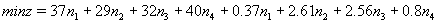subject to the constraints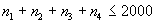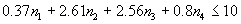( units of loss in the Sugarcane is permitted)

where n1 ≥ 0 , n2 ≥ 0 , n3 ≥ 0 , n4 ≥ 0

Then the above LPP becomes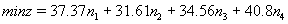subject to the constraints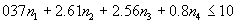where n1 ≥ 0 , n2 ≥ 0 , n3 ≥ 0 , n4 ≥ 0

This problem gives the solution for the number of units of sugarcane to be transported to get the minimum transportation cost as well as with the minimnum loss in sugarcane .

Modes and assignment of routes in the transportation of sugarcane

Usually, the transportation of sugarcane from the field to the factory is carried out either by Lorries or by Bullock carts or by the Tractors. Here, the origin is known as the fields where the sugarcane is cultivated and the destination is the factory where the processing of sugarcane is made. In this case, there are many fields, in and around the factory, and only one factory situated near by areas. The routes are divided in to three main categories and are given below and they are seggregated based on the distances of the fields to the factory.

[i] Short distance routes.

[ii] Medium distance routes.

[iii] Long distance routes.

The mode of transportation of sugarcane is done based on the above classification in order to minimize the total transportation cost and the travelling time of the vehicle. If the travelling time of the vehicle is minimum, there will be a possibility of reducing total time in the total processing of sugarcane. For the minimum distance routes, Bullock carts can be used. This takes minimum time and also the minimum cost of transportation. For the medium distance routes, Tractors can be used. This takes more cost than the above mode of transportation and it saves time, it carries little more weight than the Bullock carts. The long distance routes use , preferably, Lorries. Since, it carries maximum weight and minimum time of transportation because of high speed and transportation cost also is minimum as compared to the above routes and the modes of transportation.

Let A, B, C, D,.....be the fields (orgins) and the possible routes are specified as below.

The above transportation method (discussed earlier) is used for getting the transportation cost including the quality of the route (for each of the routes discussed in the Table 2). Along with the cost of transportation, the transportation time between the origin and the destination is also taken into account. Let this two dimensional function may be assumed as Trans (C,T) where C indicates the cost of transportation and T represents the time required to transport the sugarcane along the route specified.

 S.No Fields Routes 1. A A1 , A2 ,.... 2. B B1 , B2 ,.... 3. C C1 , C2 ,.... . . ..... . . ..... . . .....

Table 2: Fields and the possible routes.

Let D1and D2 be the distances fixed by the factory to classify the zones and the mode of transportation. The separation is as follows.

a. If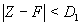where Z is the field and F is the factory. In this case, Bullock carts are used. Since, it has minimum distance and minimum cost of transportation.

b. If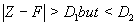, tractors are used becuase of more distance.

and

c. If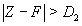, then the last mode of transportation is used. Since the lorries can transport maximum load as compared to the other two and can travel a maximum distance. The above can be put in the table as below.

For the above zones (Table 3), the rate of reduction of Sugar cane (juice content) while transporting the Sugarcane to the factory from the field is calculated. The reduction of juice content in the Sugarcane is depending on the following factors.

a. Weight of the Sugarcane loaded in the vehicle.

b. Speed of the vehicle.

c. Road condition through which the transportation is made.

 S.No. Distance Zone Vehicle 1. D1and D2 3 Lorry

Table 3: The zones and the corresponding vehicles used for the transportation.

By considering the above three factors, let the variables to the above three be assumed as Y, Z and W. These three are considered to be independent variables and the reduction of juice content in the Sugarcane is assumed to be a dependent variable and let it be assumed as X. Then the above variables are correlated using the techniques of multiple Regression analysis and it is given below.

X = A+ BY +CZ + DW

Where the constants A,B,C and D are derived from the normal equations and are given below.

ΣX = NA+ BΣY +CΣZ + DΣW

ΣXY = AΣY + BΣY 2 +CΣZY + DΣWY

ΣXZ = AΣZ + BΣYZ +CΣZ 2 + DΣWZ

ΣXW = AΣW + BΣYW +CΣZW + DΣW2

Then the rate of change in the juice content of the Sugarcane with respect to the weight of the cane loaded in the vehicle , Speed of the vehicle and the road conditions will also be given below.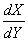- rate of reduction of juice content with respect to weight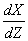- rate of reduction of juice content with respect to Speed of the vehicle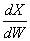- rate of reduction of juice content with respect to road conditions.

So, to reduce loss in the juice of the Sugarcane during transportation , the above points can be considered.

Numerical example: With reference to Zone-3, the experiment is done (Table 4). For the above data (Table 4), the calculation using normal equations is done in the following table (Table 5).

 S.No. X Y Z W Loss in the Jiuce Weight of Cane Speed of Road condition content in the Cane loaded in the Vehicle vehicle (in Litres) (in Kilograms) (in kmph) (Heights in cms) 1 4.5 15 60 6 2 4 15 40 15 3 1.2 13 60 4 4 1 13 60 0 5 2.3 14 50 10 6 2.8 14 35 20 7 5.1 15 45 20 8 2.3 15 40 5 9 4.9 13 55 10 10 2.8 12 45 2 11 3.9 16 60 6 12 2 12 60 2

Table 4: Experiment.

 S.No. X Y Z W XY Y2 YZ XZ YW XW Z2 W2 ZW 1 4.5 15 60 6 67.5 225 900 270 90 27 3600 36 360 2 4 15 40 15 60.0 225 600 160 225 60 1600 225 600 3 1.2 13 60 4 15.6 169 780 72 52 4.8 3600 16 240 4 1 13 60 0 13 169 780 60 0 0 3600 0 0 5 2.3 14 50 10 32.2 196 700 115 140 23 2500 100 500 6 2.8 14 35 20 39.2 196 490 98 280 56 1225 400 700 7 5.1 15 45 20 76.5 225 675 229.5 300 102 2025 400 900 8 2.3 15 40 5 34.5 225 600 92 75 11.5 1600 25 200 9 4.9 13 55 10 63.7 169 715 269.5 130 49 3025 100 550 10 0.8 12 45 2 9.6 144 540 36 24 1.6 2025 4 90 11 3.9 16 60 6 62.4 256 960 234 96 18 3600 36 360 12 2 12 60 2 24.0 144 720 120 24 4 3600 4 120

Table 5: Calculation for using normal equations.

Adding all of the above data along coloumn wise, the following results are obtained.

ΣX = 34.8 , ΣY =167 , ΣZ = 610 , ΣW =100 , ΣXY = 498.2 , ΣY2 = 2343 ΣYZ = 8460 ,ΣXZ =1756 , ΣYW =1436 ,ΣXW = 356.9 , ΣZ 2 = 32000 , ΣW2 =1346 ΣZW = 4620

Then the normal equations are

34.8 =12A+167B + 610C +100D

498.2 = 34.8A+ 2343B + 8460C +1436D

1756 = 610A+ 8460B + 32000C + 4620D

356.9 =100A+1436B + 4620C +1346D

Solving the above equations, we get the values of the constants A, B,C and D and are given below.

A = −0.005371, B = 2.43128 , C = −0.43963 , D = −1.02985

The regression equation which relates all of the above variables is given by

X = −0.005371+ 2.43128Y − 0.43963Z −1.02985W

The rate of change of juice content withe respect to the above variables are given below.= 2.43128= −0.43963= −1.02985

This calculation helps the vehicle owner to prepare for the loss occured during transportation and if it is happened, the loss can also be calculated.

The same calculation is also be made to the rest of the two zones1 and 2.

Cost involved in the accidents of vehicles during the transportation of sugarcane

In today’s context, the transportation is very important for a country’s economy because it is used for the mobility of both goods and persons. Every day a great number of vehicles carrying sugarcanes to the factory. Due to the high volume of road traffic and the high density of population, the possibility of accident is also more. So, while transporting the sugarcane, one has to decide the possibilities of accidents and prevention of accidents. One should make ready for the losses due to the accidents. This situation is modelled mathematically and it is given below.

The most general model for the expected number of vehicles meet with accidents E(λ ) is given by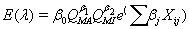where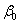- intercept to be estimated.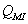- traffic flow on the minor roads.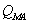- traffic flow on the major roads.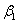- effect of traffic flow on the major roads, on the number of accidents.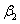- effect of traffic flow on the minor roads, on the number of accidents.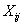- vector of explanatory variables j ,other than the traffic flow on intersection i.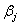- regression coefficient representing the effect of the jth explanatory variable, other than the traffic flow.

Here, the variables other than the traffic flow are assumed below.

i . Driver’s fault in making mistakes. This includes drink and drive possibility, talking with some body using mobile phone etc.,

ii . Road congestion due to the dense population of people and the vehicles.

iii .Due to overloaded vehicle

iv . Due to problems in the vehicle

v . Due to hitting the vehicle by the other vehicle

vi . Environmental conditions

Using the conditions, the number of vehicles met with the accidents may be identified. Then the probability of loss to the vehicle is defined as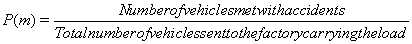ie.,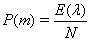where N denotes the total number of vehicles sent to the factory. Due to accident, there are some losses calculated and are given below:

(i) . loss of vehicle

(ii) . loss of life of the driver

(iii) . loss due to the damage in the road

(iv) . loss of other’s life who are affected in the accident

(v) . loss of materials which are carried in the vehicle

Let α1 , α2 , α3 , α4 , α5 be the proportions of the losses of the above cases and let C1 , C2 , C3 , C4 and C5 be the cost of one unit of above situations respectively.

Then the total loss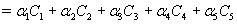In this case, we are interested to know the loss only to the sugarcane materials which is carried in the vehicle.

It is given by Loss of the sugarcane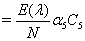#### Conclusion

This paper has overviewed the major developments in the mathematical economic modeling of transportation and has also identified some of the significant connections between the quality of the road, possibilities of the accidents happened and their corresponding losses also have been discussed. This type of conclusion also need to be done to avoid unnecessary losses to the product as well as the transportation cost.

#### Acknowledgements

The author acknowledges Professor Dr. V. Navaneethakumar, for his immense help in developing this article. The author also acknowledges the help given by the officials of Bannari Amman Sugars Limited, for providing the author with the adequate data to render the numerical example of this article.

#### References

Select your language of interest to view the total content in your interested language

### Article Usage

• Total views: 6426
• [From(publication date):
July-2012 - Aug 25, 2019]
• Breakdown by view type
• HTML page views : 2468
• PDF downloads : 3958

## Post your commentCan't read the image? click here to refresh
###### Peer Reviewed Journals

Make the best use of Scientific Research and information from our 700 + peer reviewed, Open Access Journals

International Conferences 2019-20

Meet Inspiring Speakers and Experts at our 3000+ Global Annual Meetings

Top